# Resources and Materials

This site lists resources, materials and tutorials on methods related to the research of the SPP 2171.

• # Lectures

Available lectures:

Lecture "Introduction to Nonequilibrium Thermodynamics - Onsager's variational principle"
The lecture gives a basic introduction to phenomenological nonequilibrium thermodynamics with the aim to show how typical kinetic equations in gradient dynamics form (that are e.g., employed in Soft Matter science) are derived employing Onsager’s variational principle.

Lecture "Cahn-Hilliard-type phase-transition dynamics"
The lecture gives a basic introduction to the archetypical case of a conserved gradient dynamics, i.e., Cahn-Hilliard-type models as obtained in phenomenological nonequilibrium thermodynamics with the help of Onsager’s variational principle.

Lecture "Allen-Cahn-type phase-transition dynamics"
The lecture gives a basic introduction to the archetypical case of a nonconserved gradient dynamics, i.e., Allen-Cahn-type models as obtained in phenomenological nonequilibrium thermodynamics with the help of Onsager’s variational princi

Lecture "Introduction to Numerical Continuation"
The lecture introduces in a step-by-step manner the basic concept of pseudo arclength path-continuation in a form suitable for advanced Bachelor students, Master students and beginning PhD students of the natural sciences and other interested people.

• # Software

Swalbe.jl
Simple Julia Lattice Boltzmann Solver for Thin Liquid Films and Droplets

Electronic Laboratory Notebook: A lazy approach
Electronic Lab Book Software for individuals and small groups of researchers in data-driven deductive research. The book generates itself out of notes and files from one or several experiments and is then presented as a Django-based website.

• # Data and materials on Zenodo

The SPP 2171 encourages the publication of research data and supplementary materials to the open-access repository Zenodo.

On Zenodo, we have created the SPP 2171 Community that should group all data repositories related to the SPP 2171.

• # Benchmarks

Barely any scientific result can be obtained without the use of advanced scientific methods. In order to improve the quality of research, the SPP 2171 promotes a strong scientific communication and collaboration focused on methods, techniques, and source data. Specifically, we would like to strengthen the quality of methods used with benchmarks, i.e., comparing the results obtained from different techniques on standardized experiments or scenarios.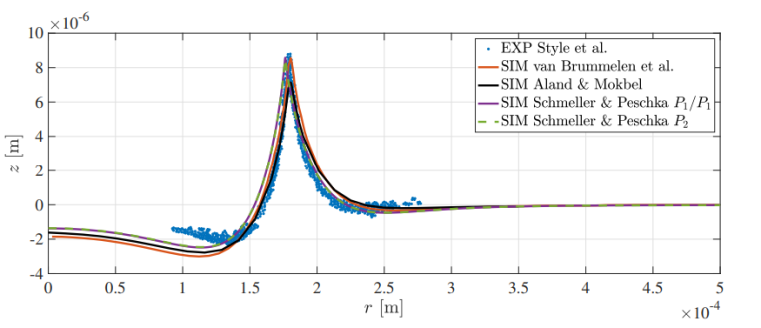Benchmark comparison of the wetting ridge of a stationary axisymmetric droplet on viscoelastic Neo-Hookean substrate© Peschka & Schmeller
• # Tutorials

• Overview of available auto07p Tutorials
The text briefly introduces the "Münsteranian Torturials on Nonlinear Science", a series of hands-on tutorials on the practical application of numerical path-continuation methods for problems in soft matter and pattern formation. A recommended sequence of working through the tutorials is laid out and for each tutorial an abstract is given.
• Steady drop and film states on a horizontal homogeneous substrate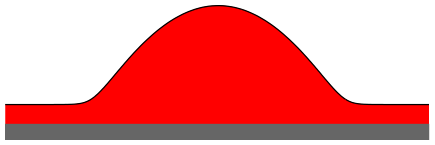This tutorial explores an equation for steady drop-and-hole solutions derived from the dimensionless thin film (or lubrication) equation. You will calculate steady solution of the equation by continuation in a number of different control parameters (domain size, mean height).
• Sliding drops modelled by a thin film equation for a liquid layer or drop on an inclined homogeneous substrate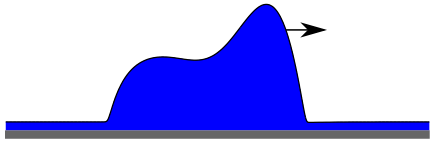In this tutorial a dimensionless thin film equation closely related to the one in DROP is used. In this case a lateral driving force is included and solutions for sliding drops on an inclined plane are examined. You will calculate steady solutions [in the comoving frame], in particular, drops moving at a constant velocity. The main treated control parameter is the inclination angle.
• Steady states of a thin film equation for a liquid layer or drop on a horizontal heterogeneous substrate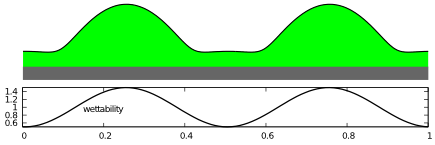In this tutorial the dimensionless thin film equation used in the tutorial DROP is adapted to a substrate of varying wettability. In particular, the long-range contribution of the disjoining pressure is sinodally modulated. You will calculate steady solutions and their bifurcations that emerge when using the heterogeneity strength as control parameter.
• Linear stability of steady solutions of a thin film equation for a horizontal homogeneous substrate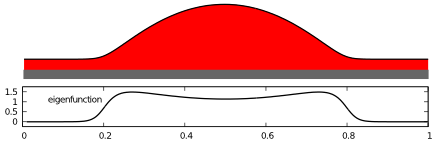This tutorial calculates the same steady solutions as the tutorial DROP but this time together with their (1d and 2d) linear stability with respect to time-dependent perturbations. You will calculate a dispersion relation (growth rate over transversal wave number) and continue solutions and their stability in various parameters.
• Steady drops on a heterogeneous substrate under lateral driving
This tutorial explores steady drops on a surface with heterogeneous wettability under the influence of lateral driving. You will calculate these steady states as a function of continuation parameters: domain length, heterogeneity strength and driving parameter.